# Box "integrals of rational functions"

Here we look at integrals of the type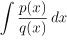, where p and q are polynomials. We have a standard procedure for them.

Algorithm for integrating ratios of polynomials.
Step 1. If the degree of the numerator is not smaller than the degree of the denominator, divide (using long division) the numerator by the denominator with a remainder. Now we will restrict our attention to that remainder, which is a ratio of polynomials that already has smaller degree of the numerator than the degree of the denominator.
Step 2. Factor the denominator as far as possible, that is, into a product of powers of linear factors and powers of irreducible (not further factorable) quadratic factors.
Step 3. Decompose the ratio of polynomials into a sum of partial fractions.
Step 4. Integrate those partial fractions.

Before we look closer at these steps, we remark that it is a good idea to first check whether the numerator happens to be (up to a multiplicative constant) equal to the derivative of the denominator. If true, then the integral can be easily solved by substitution and the above long procedure is not needed.

Step 2 is a problem from linear algebra. Often the denominator already is factored, sometimes it is enough to factor something out, sometimes it is necessary to find roots, which is most often done using the quadratic formula or guessing (when the degree is higher). More details can be found in Polynomials in Functions - Theory - Elementary function. How one can quess factorization of a quadratic polynomial we wrote this note.

Step 3 is in fact an independent topic, therefore we dedicated a special page for it.

Step 4: We see that in order to be able to integrate rational functions, all we need to learn is how to integrate the partial fractions, in other words, evaluate the following two types of integrals: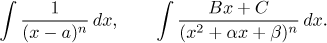For both we have a standard procedure.

Partial fraction with linear term: These can be integrated easily using an obvious linear substitution. For n = 1 we have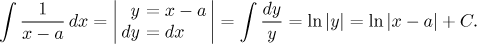For n ≠ 1 we get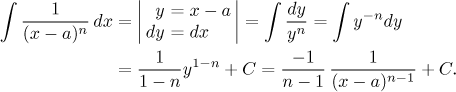Therefore problems where there are no quadratic factors present in q(x) are very easy, both the decomposition and the following integration are simplest possible.

Partial fractions with quadratic terms: These can range from simple to longer, depending on how complicated the term is. We start with the easiest case and then show how the more complicated terms transform into those simple ones. General strategy is that if a term has x in the numerator, then we use it to set up a quadratic substitution, which is the easy case. If there si a constant in the numerator, then the integral is transformed to the integral leading to arc tangent.

a) The basic type with a constant in the numerator is easily transformed into an elementary integral using a simple indirect substitution.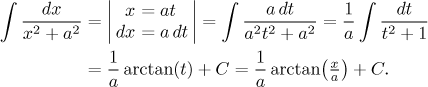Some people prefer to memorize this integral. There is also an alternative way to do the transformation, first one factors out a from the denominator and then uses direct substitution, see here.

b) The basic type with x in the numerator is easily solved using a quadratic substitution.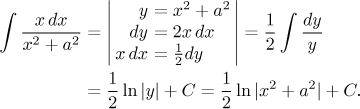A term with both x and a constant in the numerator can be easily split into two integrals that we already know how to handle.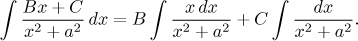c) The term with x in the numerator is not really a problem, quadratic the substituion still works. For n > 1 we get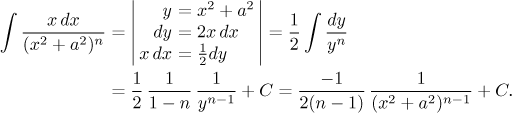d) The term with a constant in the numerator and a higher power in the denominator is the worst of all, since there is no direct way to evaluate such an integral. Instead, one uses a special reduction formula to dectrease the power by 1 at each step until an integral leading to arc tangent is obtained. The reduction formula: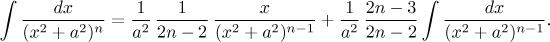If you are curious about the origin of it, click here. There is an alternative to treat such an integral as something from the box "powers of quadratics", which may be easier when n = 2 (see this problem in Solved Problems - Integrals) but in general it again leads to some reduction formula.

e) It remains to explore the most general case, when we have a general quadratic expression in the denominator. Here the procedure calls for completing square in the denominator, substitution then transforms the given integral into types that we know how to handle.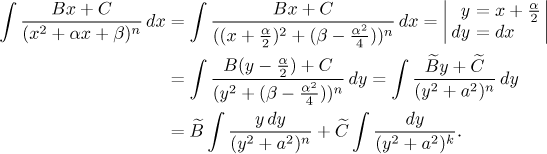One can save time by combining this procedure with substitution from a), the resulting mixed substitution at = x + α/2 creates the term t2 + 1 in the denominator right away, making successive calculations easier.

Note that the order is important, first we get rid of the linear part in the denominator and only then we split the numerator into two fractions. If we changed the order and first split the integral into two, one with x and the other with a constant in the numerator, and only then applied the substitution trick, then the integral with x would after substitution turn into an integral that has both y and a constant in its numerator, thus forcing us to split it again unnecessarily. There is an alternative algorithm that gets around this problem, some readers may find it more convenient and we describe it here. Here in Math Tutor we however stick to the procedure described above.

Note also that after completing the square we always end up with the expression y2 + A with A > 0. In general, using completion of square we could also obtain y2 − A or A − y2, but these two polynomials can be factored into linear factors, while quadratic factors in partial franctions are already irreducible and this prevents these two cases from happening here.

The procedure described above can be slightly modified to better fit particular situations. We will show a couple of such modifications in the following example.

Example: We will start evaluating the following integral. Because the degree in numerator is not lower than the degree in the denominator, we first divide with remainder and then focus on that remainder. The next step calls for decomposing its denominator, then we set up partial fractions.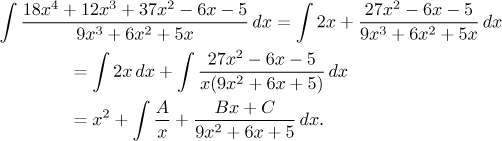Note that we do not exactly follow the decomposition as specified in theory. Properly we should have factored out the nine from the quadratic term, so that it starts with x2. But this would lead to unnecessary fractions, so we prefer to leave it as it is for a while. It can be proved that we do not do anything wrong this way, we will just have to slightly modify one of the steps later.

Finding the unknown constants is quite easy, since there are only three of them. We have one real root of multiplicity 1, so the correcponding can be obtained for free using the "cover-up" trick. The other two can be obtained for instance by the multiplying method.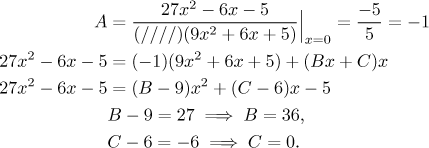Thus we get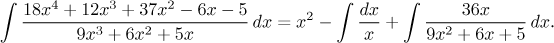The first partial fraction is linear and therefore very simple, so we will focus on the second one, which happens to be case e). First we have to complete square. Note that the constant 9 that we did not factor out does not really bother us, we include it in the completion process. Then we use the recommended mixed substitution that removes the linear part and also creates a 1 in the denominator.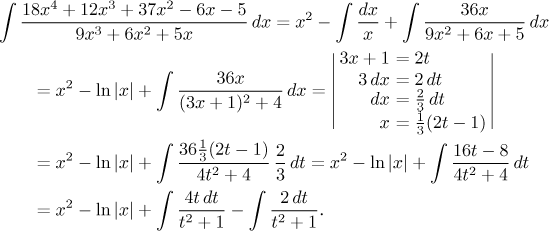Now we wasily conclude the calculation.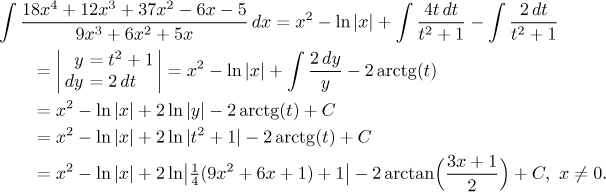It remains to make the answer nicer. Using the identity for logarithm of a product we move the one quarter away as a separate term 2ln(1/4) in the answer, but this is a constant, so we can merge it with the exosting constant C. Thus we get a more pleasing answer.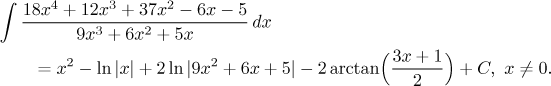Most problems in Solved Problems - Integrals lead sooner or later to partial fractions, so you have lots of examples to study, of special interest are this problem, this problem, this problem and this problem.